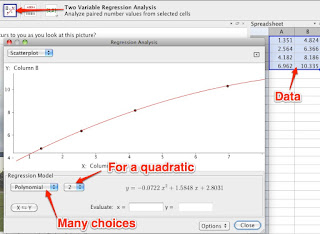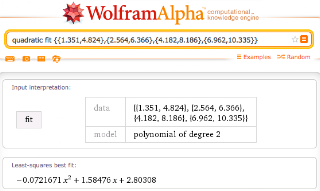## Saturday, June 16, 2012

I'm teaching a summer intermediate algebra course, and we recently had a full day of GeoGebra for quadratic functions. With GVSU's GeoGebra training coming up, it seemed like a good time to document what all we did.

I'm using a Bring Your Own Device philosophy for the class, so students are bringing TIs (82, 83, 84, 86 so far), smart mobiles, and laptops to run Desmos or GeoGebra. When we have a particularly heavy computer day, I let them know ahead, and a few extra people bring in laptops. The university runs a pretty good wireless service accessible to all students and staff.

Here's our agenda for the day:
Agenda – Class 9
Objective: quadratic regression and connections between the vertex form and standard form.

5 start up: multiplying 2 digit numbers with place value sense.
15 HW
30 Vertex investigation (GGBT - GeoGebraTube)
50 Regression opportunities (W|A, GGB, TI)
30 Projectiles
5 Classroom concerns
To do: project 1 – linear and/or quadratic math in life.
The way the class panned out, we left off projectiles for another day. The point of that is to make a strong connection between constant second difference and constant acceleration.

Some of what we were doing was to support them in the project. Here's the handout:

It helped engagement that they knew there was something for class that this supported.

The multiplication of 2 digit numbers was a connect forward piece to multiplication of binomials, by means of an area model that shows partial products and leads to the box method for multiplying polynomials.  There were no homework questions, so we spent a while on the multiplying, and then someone wanted to know why we were doing this, so we got into the box method right then and there.

I designed this sketch to have just a and b on sliders, to focus attention on those. The class had previously noticed that c in the standard form was the y-intercept, so I made that literal in the sketch to emphasize it. I gave it a dynamic window (defining x- and y-min and max interms of function values) so that the parabola would be in view. (GeoGebra note: that means that the program is constantly recomputing when values are changed, so you can't really use the trace.) The sketch has these questions on GGBTube.
This sketch helps you investigate the location of the vertex for a parabola in standard form, y=ax^2+bx+c. Since the equation will tell us y if we know x, we will concentrate on the x-coordinate of the vertex.

1) There are controls for a and b, separate from c. Why doesn't c change the left-right location of the vertex?

2) Change a and b and notice the effect on whether the vertex moves left or right. What do you notice?

3) Our goal is to find a rule or formula for the vertex's x-coordinate in terms of a and b. How can you collect data that will help us determine the formula?

Extension: what else could you investigate with this sketch?

The vertex investigation led to a good bit of mathematical reasoning. They noticed that when a increased, the vertex moved to the right, unless b was negative in which case it moved to the left. Some discussion led them to decide that it moved closer to zero.  They noticed when b=0 that the vertex is always at x=0. They also noticed a cool parabola pattern that the vertex makes when you move the b slider.

I suggested that maybe we needed some hard data, and started recording on the board a and b vs. the x-coordinate of the vertex for what they had tried. No pattern was visible. So I suggested fixing a or b and varying the other. Now there's some interesting things to see.
(It wasn't this neat on the board.) They noticed that as b increased it got bigger ("Bigger?" I ask. "Well, more negative.") As a increased it got smaller. When a was steady, it was like a line. They noticed the -1/2 slope for when a=1. One student noticed that when b doubled, the x-coordinate doubled. Then another noticed that when a doubled, it went down by half. Then a student had the insight, "oh, I have it! I think... can I say?" (We've been working a bit on being a no-spoilers class.) Is it -b/2a?  Seemed to work with all the data we had.

Steve's Rule: x-coordinate of the vertex = -b/2a.

When students reflected on this investigation (jot down one thing you want to remember) many noted the formula, but some remarked on collecting the data and the trends in a and b as it affected the vertex.

Next I wanted to give them some practice on regression. Starting with the sketch Dan and the Ball,
which, of course, is from Dan Meyer's photo composite.  Students pretty naturally want to know if it goes in. Most think it does when you take a vote.On this sketch you can use the red point to gather data. We talked about entering the data in the spreadsheet, from which you can use the two-variable data tool.You can also use Wolfram|Alpha to do the regression, or a TI. Unfortunately Desmos doesn't have regression yet. W|A is surprisingly finicky about the phrasing you use. For example it prefers "fit" over "regression."Students were very successful with this, and I had them move on to their choice of activity.

Several did the bridge problem, which looks at whether a bridge is a parabola or a catenary. They were bold about exploring, despite the many overheard comments about not having any idea what was going on with the catenary. Several also gave the bouncing ball sketch a try, which allowed them to see the effect of constant a on different parabolas. (OK, I'm also just pretty happy with the simulation.)By that point many were looking for other things on their own. Someone found this great world record motocross time lapse shot, among others.From tifr
I made up a guide to importing and using images in GeoGebra.

One GeoGebraTube tip that I picked up from this much student use in class was to remember to change the vertical display size of a sketch if you are adding the menu bar, tool bar or input bar. About 200 pixels is enough for all three.
You get to that screen by being logged in and choosing the Edit option on the teacher page for your sketch.

This day seemed to make (by subsequent assessment) a difference in their technical ability to do regression, their understanding of the vertex formula and use of the quadratic formula, understanding of some of the features of parabolas. They also have been working with a great amount of independence on their projects.  Which I am excited to see on Monday!